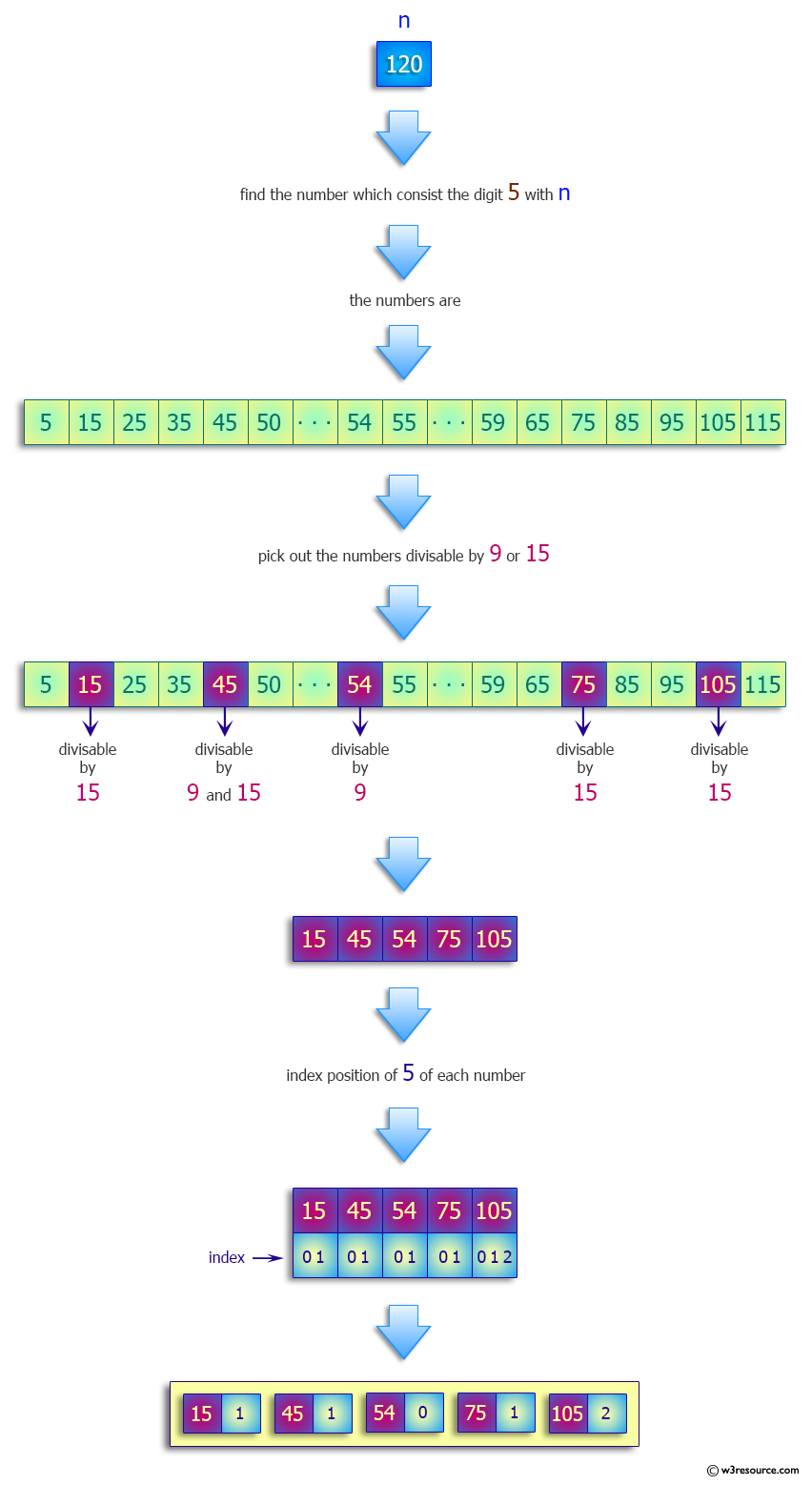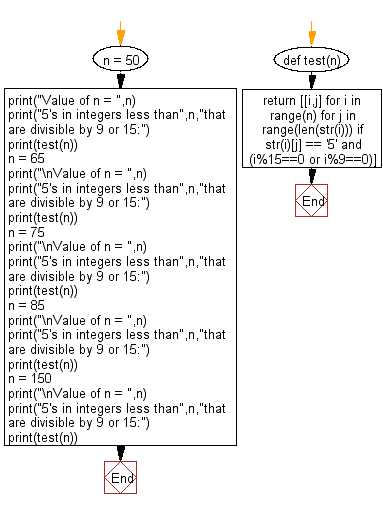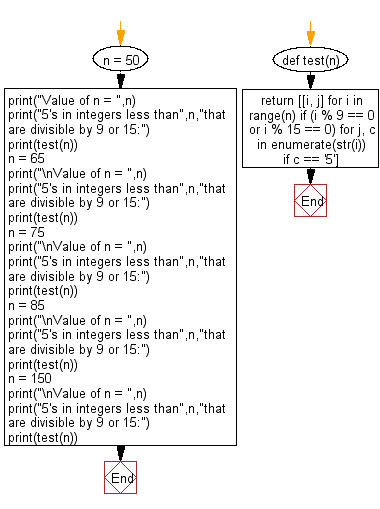﻿ Python: Find all 5's in integers less than n that are divisible by 9 or 15 - w3resource# Python: Find all 5's in integers less than n that are divisible by 9 or 15

## Python Programming Puzzles: Exercise-68 with Solution

Write a Python program to find all 5's in integers less than n that are divisible by 9 or 15.

```Input:
Value of n =  50
Output:
[[15, 1], [45, 1]]
Input:
Value of n =  65
Output:
[[15, 1], [45, 1], [54, 0]]
Input:
Value of n =  75
Output:
[[15, 1], [45, 1], [54, 0]]
Input:
Value of n =  85
Output:
[[15, 1], [45, 1], [54, 0], [75, 1]]
Input:
Value of n =  150
Output:
[[15, 1], [45, 1], [54, 0], [75, 1], [105, 2], [135, 2]]
```

Pictorial Presentation:Sample Solution-1:

Python Code:

``````def test(n):
return [[i,j] for i in range(n) for j in range(len(str(i))) if str(i)[j] == '5' and (i%15==0 or i%9==0)]
n = 50
print("Value of n = ",n)
print("5's in integers less than",n,"that are divisible by 9 or 15:")
print(test(n))
n = 65
print("\nValue of n = ",n)
print("5's in integers less than",n,"that are divisible by 9 or 15:")
print(test(n))
n = 75
print("\nValue of n = ",n)
print("5's in integers less than",n,"that are divisible by 9 or 15:")
print(test(n))
n = 85
print("\nValue of n = ",n)
print("5's in integers less than",n,"that are divisible by 9 or 15:")
print(test(n))
n = 150
print("\nValue of n = ",n)
print("5's in integers less than",n,"that are divisible by 9 or 15:")
print(test(n))
``````

Sample Output:

```Value of n =  50
5's in integers less than 50 that are divisible by 9 or 15:
[[15, 1], [45, 1]]

Value of n =  65
5's in integers less than 65 that are divisible by 9 or 15:
[[15, 1], [45, 1], [54, 0]]

Value of n =  75
5's in integers less than 75 that are divisible by 9 or 15:
[[15, 1], [45, 1], [54, 0]]

Value of n =  85
5's in integers less than 85 that are divisible by 9 or 15:
[[15, 1], [45, 1], [54, 0], [75, 1]]

Value of n =  150
5's in integers less than 150 that are divisible by 9 or 15:
[[15, 1], [45, 1], [54, 0], [75, 1], [105, 2], [135, 2]]
```

Flowchart:## Visualize Python code execution:

The following tool visualize what the computer is doing step-by-step as it executes the said program:

Sample Solution-2:

Python Code:

``````def test(n):
return [[i, j] for i in range(n) if (i % 9 == 0 or i % 15 == 0) for j, c in enumerate(str(i)) if c == '5']

n = 50
print("Value of n = ",n)
print("5's in integers less than",n,"that are divisible by 9 or 15:")
print(test(n))
n = 65
print("\nValue of n = ",n)
print("5's in integers less than",n,"that are divisible by 9 or 15:")
print(test(n))
n = 75
print("\nValue of n = ",n)
print("5's in integers less than",n,"that are divisible by 9 or 15:")
print(test(n))
n = 85
print("\nValue of n = ",n)
print("5's in integers less than",n,"that are divisible by 9 or 15:")
print(test(n))
n = 150
print("\nValue of n = ",n)
print("5's in integers less than",n,"that are divisible by 9 or 15:")
print(test(n))
``````

Sample Output:

```Value of n =  50
5's in integers less than 50 that are divisible by 9 or 15:
[[15, 1], [45, 1]]

Value of n =  65
5's in integers less than 65 that are divisible by 9 or 15:
[[15, 1], [45, 1], [54, 0]]

Value of n =  75
5's in integers less than 75 that are divisible by 9 or 15:
[[15, 1], [45, 1], [54, 0]]

Value of n =  85
5's in integers less than 85 that are divisible by 9 or 15:
[[15, 1], [45, 1], [54, 0], [75, 1]]

Value of n =  150
5's in integers less than 150 that are divisible by 9 or 15:
[[15, 1], [45, 1], [54, 0], [75, 1], [105, 2], [135, 2]]
```

Flowchart:## Visualize Python code execution:

The following tool visualize what the computer is doing step-by-step as it executes the said program:

Python Code Editor :

Have another way to solve this solution? Contribute your code (and comments) through Disqus.

What is the difficulty level of this exercise?

Test your Programming skills with w3resource's quiz.

﻿

## Python: Tips of the Day

Clamps num within the inclusive range specified by the boundary values x and y:

Example:

```def tips_clamp_num(num,x,y):
return max(min(num, max(x, y)), min(x, y))
print(tips_clamp_num(2, 4, 6))
print(tips_clamp_num(1, -1, -6))
```

Output:

```4
-1
```